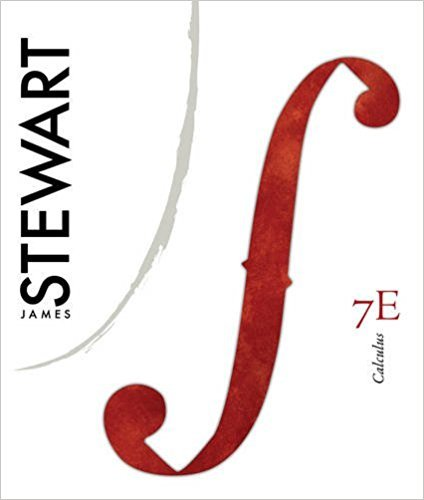×
Get Full Access to Calculus, - 7 Edition - Chapter 9 - Problem 25
Get Full Access to Calculus, - 7 Edition - Chapter 9 - Problem 25

×

# When a flexible cable of uniform density is suspendedISBN: 9780538497817 125

## Solution for problem 25 Chapter 9

Calculus, | 7th Edition

• Textbook Solutions
• 2901 Step-by-step solutions solved by professors and subject experts
• Get 24/7 help from StudySoup virtual teaching assistantsCalculus, | 7th Edition

4 5 1 314 Reviews
14
2
Problem 25

When a flexible cable of uniform density is suspended between two fixed points and hangs of its own weight, the shape of the cable must satisfy a differential equation of the form 100% y f x d2 y dx 2 k 1 dy dx 2 where is a positive constant. Consider the cable shown in the figure. (a) Let in the differential equation. Solve the resulting first-order differential equation (in ), and then integrate to find . (b) Determine the length of the cable. z dydx z y x b 0 y _b (0, a) (_b, h) (b, h)

Step-by-Step Solution:
Step 1 of 3

Step 2 of 3

Step 3 of 3

##### ISBN: 9780538497817

This full solution covers the following key subjects: cable, equation, Differential, length, determine. This expansive textbook survival guide covers 17 chapters, and 887 solutions. Since the solution to 25 from 9 chapter was answered, more than 319 students have viewed the full step-by-step answer. This textbook survival guide was created for the textbook: Calculus,, edition: 7. Calculus, was written by and is associated to the ISBN: 9780538497817. The full step-by-step solution to problem: 25 from chapter: 9 was answered by , our top Calculus solution expert on 11/10/17, 05:23PM. The answer to “When a flexible cable of uniform density is suspended between two fixed points and hangs of its own weight, the shape of the cable must satisfy a differential equation of the form 100% y f x d2 y dx 2 k 1 dy dx 2 where is a positive constant. Consider the cable shown in the figure. (a) Let in the differential equation. Solve the resulting first-order differential equation (in ), and then integrate to find . (b) Determine the length of the cable. z dydx z y x b 0 y _b (0, a) (_b, h) (b, h)” is broken down into a number of easy to follow steps, and 99 words.

Unlock Textbook Solution